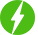# Probability Part – 2

1

Probability Part – 2, Probability.

## Course Description

1. A bag contains lemon flavoured candies only. What is the probability of getting an orange flavoured candy? How to calculate the probability of getting a lemon candy?

2. In a group of 3 students, the probability of 2 students not having the same birthday is given. How to calculate the probability of 2 students having the same birthday?

3. A bag contains red balls and black balls. How to calculate the probability that ball drawn is (i) red (ii) not red?

4. A box contains red, white and green marbles. How to calculate the probability that the marble taken out is (i) red (ii) white (iii) not green?

5. A piggy bank contains coins of different denomination. If one of the coins falls when the piggy bank is turned upside down, what is the probability that the coin (i) will be a 50 p coin (ii) will not be a Rs.5 coin?

6. A tank contains male fish and female fish. What is the probability that the fish taken out is a male fish?

7. A game of chance consists of spinning an arrow which comes to rest pointing at one of the numbers from 1 to 8. What is the probability that it will point at (i) 8 (ii) an odd number (iii) a number greater than 2 (iv) a number less than 9?

A dice is thrown once. How to find the probability of getting (i) a prime number (ii) a number lying between 2 and 6 (iii) an odd number?

We will be happy to hear your thoughtsRegister New Account
• Total (0)
0